﻿ 空实心GFRP管钢筋混凝土偏心受压构件徐变分析

# 空实心GFRP管钢筋混凝土偏心受压构件徐变分析Creep Analysis of Hollow and Solid GFRP Tube Reinforced Concrete Eccentric Compression Member

Abstract: In order to study the effect of creep on the eccentric compression members of hollow and solid GFRP tube reinforced concrete, the creep formula of hollow and solid GFRP tube reinforced con-crete was established. GFRP tube reinforced concrete eccentric compression member creep effect analysis and calculation was established, and a numerical example was calculated, analyzing the effect of eccentricity, the hollow rate, action load, the thickness of GFRP tube and strength grade of concrete to influence of eccentric compression member creep. Results of the computation showed that the creep of the eccentric compression member was increased with the increase of eccentricity, hollow rate, action load and strength grade of concrete, the decrease of the thickness of GFRP tube. The influence of hollow rate and action of the creep of GFRP tube reinforced concrete eccentric compression member was large, and eccentricity and the thickness of GFRP tube were the secondary factor. The strength grade of concrete was relatively small.

1. 引言

GFRP (Glass Fiber Reinforced Plastic，中文名玻璃纤维增强塑料)管钢筋混凝土构件是在GFRP管内设置纵向受力钢筋，再向其内部浇筑混凝土而形成的一种新型构件。随着研究的深入和应用的发展，从实心截面逐渐发展到了空心截面   。GFRP管与混凝土是物理、力学性能截然不同的两种材料，为此，在长期使用过程中，由于受到时间、周围环境等变化的影响，混凝土会发生收缩与徐变，从而导致了GFRP管和混凝土发生内力重分布等。GFRP管钢筋混凝土构件主要用于轴心受压的结构中，但在实际应用中，由于混凝土的不均匀性、荷载位置的不确定性及施工偏差，轴心受压状态几乎不可能出现，绝大多数的受压构件处于偏心受压状态。国内外一些学者、专家对GFRP管钢筋混凝土构件的偏心受压性能进行研究  - ，但对其徐变效应的研究并不多见。本文对空、实心GFRP管钢筋混凝土偏心受压构件的初始状态进行分析，求得核心混凝土的初始应力以及偏心受压构件的徐变计算公式。编制空、实心GFRP管钢筋混凝土偏心受压构件徐变计算程序，分析了主要参数对其徐变性能的影响。

2. 偏心受压构件初始状态受力分析

1) GFRP管与混凝土之间无相对滑移；

2) 截面应变符合平截面假定；

3) 不考虑剪切变形的影响；

4) 构件两端为铰接，且挠曲线为正弦半波曲线。

2.1. 受力分析

${\sigma }_{f}={N}_{f}\left(\frac{1}{{A}_{f}}+\frac{e{r}_{f}}{{I}_{f}}\right)$ (1)

${\sigma }_{c}={N}_{c}\left(\frac{1}{\left({A}_{c}-{A}_{ch}\right)}+\frac{e{r}_{f}}{{I}_{c}}\right)$ (2)

$M=N\left({e}_{0}+f\right)$$e={e}_{0}+f=\eta {e}_{0}$

2.2. 紧箍应力分析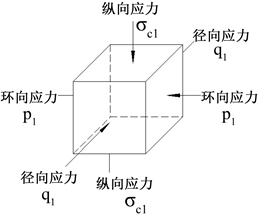Figure 1. Eccentric core concrete stress

${\epsilon }_{c1}=\frac{1}{{E}_{c}}\left[{\sigma }_{c1}-{\mu }_{c}\left({p}_{1}+{q}_{1}\right)\right]$ (3)

${\epsilon }_{c2}=\frac{1}{{E}_{c}}\left[{q}_{1}-{\mu }_{c}\left({\sigma }_{c1}+{p}_{1}\right)\right]$ (4)

${\epsilon }_{c3}=\frac{1}{{E}_{c}}\left[{p}_{1}-{\mu }_{c}\left({\sigma }_{c1}+{q}_{1}\right)\right]$ (5)

GFRP管的应力状态，见图2Figure 2. The stress of GFRP tube

${\sigma }_{f3}=-\frac{{r}_{f}}{{t}_{f}}{q}_{1}$

${\epsilon }_{f1}=\frac{{\sigma }_{f1}}{{E}_{f1}}-\frac{{\mu }_{f3}}{{E}_{f3}}{\sigma }_{f3}$ (6)

${\epsilon }_{f2}=-\frac{{\mu }_{f1}}{{E}_{f1}}{\sigma }_{f1}-\frac{{\mu }_{f3}}{{E}_{f3}}{\sigma }_{f3}$ (7)

${\epsilon }_{f3}=\frac{{\sigma }_{f3}}{{E}_{f3}}-\frac{{\mu }_{f1}}{{E}_{f1}}{\sigma }_{f1}$ (8)

$\frac{1}{{E}_{c}}\left[{\sigma }_{c1}-{\mu }_{c}\left({p}_{1}+{q}_{1}\right)\right]=\frac{{\sigma }_{f1}}{{E}_{f1}}-\frac{{\mu }_{f3}}{{E}_{f3}}{\sigma }_{f3}$

$\frac{1}{{E}_{c}}\left[{q}_{1}-{\mu }_{c}\left({\sigma }_{c1}+{p}_{1}\right)\right]=-\frac{{\mu }_{f1}}{{E}_{f1}}{\sigma }_{f1}-\frac{{\mu }_{f3}}{{E}_{f3}}{\sigma }_{f3}$

$\frac{1}{{E}_{c}}\left[{p}_{1}-{\mu }_{c}\left({\sigma }_{c1}+{q}_{1}\right)\right]=\frac{{\sigma }_{f3}}{{E}_{f3}}-\frac{{\mu }_{f1}}{{E}_{f1}}{\sigma }_{f1}$

${q}_{1}={n}_{1}{\sigma }_{c1}$ (9)

${p}_{1}={n}_{2}{\sigma }_{c1}$ (10)

${m}_{1}=\frac{{\mu }_{c}\left({\mu }_{f1}+1\right)}{{E}_{c}}+\frac{{\mu }_{f3}\left({\mu }_{f1}+1\right){r}_{f}}{{E}_{f3}{t}_{f}}$${m}_{2}=\frac{\frac{1+{\mu }_{c}}{{E}_{c}}}{\frac{1-{\mu }_{c}}{{E}_{c}}+\frac{\left(1-{\mu }_{f3}\right){r}_{f}}{{E}_{f3}{t}_{f}}}$

${\sigma }_{f1}=\left(\frac{1-{\mu }_{c}{n}_{2}-{\mu }_{c}{n}_{1}}{{E}_{c}}-\frac{{\mu }_{f3}{r}_{f}{n}_{2}}{{E}_{f3}{t}_{f}}\right){E}_{f1}{\sigma }_{c1}$(11)

2.3. 初始应力计算

$N={N}_{f}+{N}_{c}+{N}_{s}$

${N}_{s}=\frac{{A}_{s}}{{A}_{c}-{A}_{ch}}\cdot \frac{{E}_{s}}{{E}_{c}}{N}_{c}$

${N}_{f}=\gamma \left(\frac{1-{\mu }_{c}{n}_{2}-{\mu }_{c}{n}_{1}}{{E}_{c}}-\frac{{\mu }_{f3}{r}_{f}{n}_{2}}{{E}_{f3}{t}_{f}}\right){E}_{f1}{N}_{c}$ (12)

${N}_{c}=\frac{N}{1+\gamma \left(\frac{1-{\mu }_{c}{n}_{2}-{\mu }_{c}{n}_{1}}{{E}_{c}}-\frac{{\mu }_{f3}{r}_{f}{n}_{2}}{{E}_{f3}{t}_{f}}\right)+\frac{{A}_{s}}{{A}_{c}-{A}_{ch}}\cdot \frac{{E}_{s}}{{E}_{c}}}$ (13)

GFRP管最大受压纤维处初始应力为：

${\sigma }_{f0}=\frac{\left(\frac{1-{\mu }_{c}{n}_{2}-{\mu }_{c}{n}_{1}}{{E}_{c}}-\frac{{\mu }_{f3}{r}_{f}{n}_{2}}{{E}_{f3}{t}_{f}}\right){E}_{f1}\left(\frac{1}{{A}_{c}-{A}_{ch}}+\frac{er}{{I}_{c}}\right)}{1+\gamma \left(\frac{1-{\mu }_{c}{n}_{2}-{\mu }_{c}{n}_{1}}{{E}_{c}}-\frac{{\mu }_{f3}{r}_{f}{n}_{2}}{{E}_{f3}{t}_{f}}\right)+\frac{{A}_{s}}{{A}_{c}-{A}_{ch}}\cdot \frac{{E}_{s}}{{E}_{c}}}N$ (14)

${\sigma }_{c0}=\frac{N}{1+\gamma \left(\frac{1-{\mu }_{c}{n}_{2}-{\mu }_{c}{n}_{1}}{{E}_{c}}-\frac{{\mu }_{f3}{r}_{f}{n}_{2}}{{E}_{f3}{t}_{f}}\right)+\frac{{A}_{s}}{{A}_{c}-{A}_{ch}}\cdot \frac{{E}_{s}}{{E}_{c}}}\left(\frac{1}{{A}_{c}-{A}_{ch}}+\frac{er}{{I}_{c}}\right)$(15)

3. 偏压构件徐变理论计算公式

$\left(1+\frac{{A}_{s}}{{A}_{c}-{A}_{ch}}\frac{{E}_{s}}{{E}_{c}}\right){N}_{c}^{c}+{N}_{f}^{c}=0$ (16)

${\sigma }_{c1}^{c}={N}_{c}^{c}\left(\frac{1}{{A}_{c}-{A}_{ch}}+\frac{e{r}_{f}}{{I}_{c}}\right)$

${\sigma }_{c1}^{c}={N}_{f}^{c}\left(\frac{1}{{A}_{f}}+\frac{e{r}_{f}}{{I}_{f}}\right)$

${\sigma }_{f1}^{c}=-\frac{1}{\gamma }\left(1+\frac{{A}_{s}}{{A}_{c}-{A}_{ch}}\cdot \frac{{E}_{s}}{{E}_{c}}\right){\sigma }_{c1}^{c}$ (17)

GFRP管发生徐变时，其应变变化为：

${\epsilon }_{f1}^{c}=\frac{{\sigma }_{f1}^{c}}{{E}_{f1}}+\frac{{\mu }_{f3}}{{E}_{f3}}\frac{r}{t}\Delta {q}_{1}$

${\epsilon }_{c1}^{c}=\left({\sigma }_{c0}+{\sigma }_{c1}^{c}\right){c}_{1}$

${c}_{1}=c\cdot \left[1-{\mu }_{cp,1}\left({n}_{1}+{n}_{2}\right)\right]$ (18)

$c=\left[1.51\left(1-{\text{e}}^{-2.7\left(t-{t}_{0}\right)}\right)+3.34\left(1-{\text{e}}^{-0.14\left(t-{t}_{0}\right)}\right)+2.17\left(1-{\text{e}}^{-1.15\left(t-{t}_{0}\right)}\right)+8.85\left(1-{\text{e}}^{-0.015\left(t-{t}_{0}\right)}\right)\right]×{10}^{-6}$

${\mu }_{cp,1}=0.16-\frac{0.074}{{n}_{1}+{n}_{2}}+\frac{0.028}{{\left({n}_{1}+{n}_{2}\right)}^{2}}$

GFRP管径向应变变化为：

${\epsilon }_{f2}^{c}=-\frac{{\mu }_{f1}}{{E}_{f1}}{\sigma }_{f1}^{c}+\frac{{\mu }_{f3}}{{E}_{f3}}\frac{r}{t}\Delta {q}_{1}$

${c}_{2}=c\left(1-{\mu }_{cp,2}\frac{1+{n}_{2}}{{n}_{1}}\right)$ (19)

${\mu }_{cp,2}=0.16-\frac{0.074}{\frac{1+{n}_{2}}{{n}_{1}}}+\frac{0.028}{{\left(\frac{1+{n}_{2}}{{n}_{1}}\right)}^{2}}$

${\epsilon }_{f2}^{c}={\epsilon }_{c2}^{c}$

$-\frac{{\mu }_{f1}}{{E}_{f1}}{\sigma }_{f1}^{c}+\frac{{\mu }_{f3}}{{E}_{f3}}\frac{r}{t}\Delta {q}_{1}=\left({q}_{1}+\Delta {q}_{1}\right){c}_{2}$

${\epsilon }_{f2}^{c}={\epsilon }_{c2}^{c}$

$\frac{{\sigma }_{f1}^{c}}{{E}_{f1}}+\frac{{\mu }_{f3}}{{E}_{f3}}\frac{r}{t}\Delta {q}_{1}=\left({\sigma }_{c0}+{\sigma }_{c1}^{c}\right){c}_{1}$

$\Delta {q}_{1}=\frac{{n}_{1}{c}_{2}{\sigma }_{co}-\frac{{\mu }_{f1}}{{E}_{f1}}\frac{1}{\gamma }\left(1+\frac{{A}_{s}}{{A}_{c}-{A}_{ch}}\frac{{E}_{s}}{{E}_{c}}\right){\sigma }_{c1}^{c}}{\frac{{\mu }_{f3}}{{E}_{f3}}\frac{{r}_{f}}{{t}_{f}}-{c}_{2}}$ (20)

${\sigma }_{c1}^{c}=-\frac{{c}_{1}-{n}_{1}{c}_{2}-\frac{{E}_{f3}}{{\mu }_{f3}}\frac{{t}_{f}}{{r}_{f}}{c}_{1}{c}_{2}}{\frac{{\mu }_{f1}}{{E}_{f1}}\frac{1}{\gamma }{n}_{3}+{c}_{1}+\frac{1}{\gamma {E}_{f1}}{n}_{3}-\frac{{E}_{f3}}{{\mu }_{f3}}\frac{{t}_{f}}{{r}_{f}}{c}_{1}{c}_{2}-\frac{{E}_{f3}}{{\mu }_{f3}}\frac{{t}_{f}}{{r}_{f}}\frac{1}{\gamma {E}_{f1}}{n}_{3}{c}_{2}}{\sigma }_{c0}$ (21)

${\epsilon }_{c}^{c}=\left({\sigma }_{c0}+{\sigma }_{c1}^{c}\right)c$(22)

4. 计算分析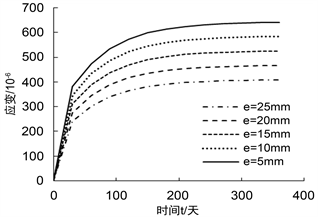Figure 3. The influence curve of eccentricity

1) 偏心距的影响

2) 空心率的影响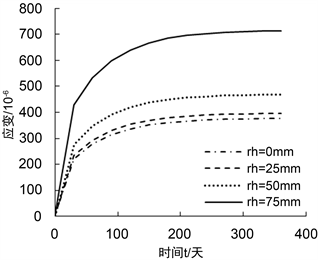Figure 4. The influence curve of hollow rate on creep

3) 作用荷载的影响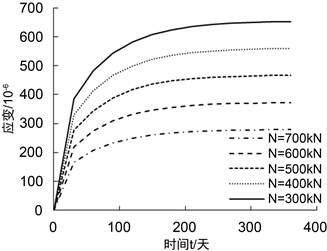Figure 5. The influence curve of action load on creep

4) GFRP管管壁厚度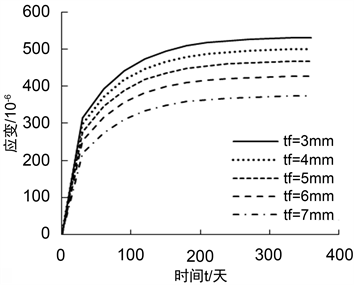Figure 6. The influence curve of the thickness of GFRP tube on creep

5) 混凝土强度等级的影响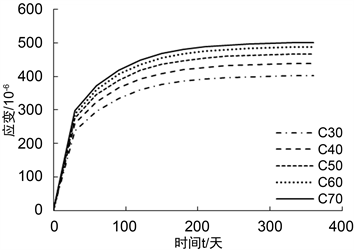Figure 7. The influence curve of concrete strength grade on creep

5. 结语

1) 对空、实心GFRP管钢筋混凝土偏心受压构件进行分析，建立同时适用于实心和空心GFRP管钢筋混凝土偏心受压构件的徐变公式。

2) 编制徐变分析程序，分析了偏心距、空心率、作用荷载、GFRP管管壁厚度及混凝土强度等级等参数对GFRP管钢筋混凝土偏心受压构件徐变的影响。

3) GFRP管钢筋混凝土偏心受压构件的徐变随偏心距的增大、空心率的增大、作用荷载的增大、GFRP管管壁厚度的减小及混凝土强度等级的提高而增大。空心率和作用荷载对GFRP管钢筋混凝土偏心受压构件徐变的影响较大，偏心距和GFRP管管壁厚度的影响次之，混凝土强度等级的影响相对较小。

NOTES

*通讯作者。

 王连广, 陈百玲. GFRP管及钢管型钢混凝土结构[M]. 沈阳: 辽宁科学技术出版社, 2013.

 汪子豪. FRP–混凝土–高强钢实心双管柱的试验研究[D]: [博士学位论文]. 杭州: 浙江大学, 2017.

 Ahmad, I., Zhu, Z.Y. and Mirmiran, A. (2008) Behavior of Short and Deep Beams Made of Concrete-Filled Fiber-Reinforced Polymer Tubes. Journal of Composites for Construction, 12, 102-110.
https://doi.org/10.1061/(ASCE)1090-0268(2008)12:1(102)

 肖建庄, 刘胜. 钢管/GFRP管约束再生混凝土柱偏心受压试验[J]. 建筑科学与工程学报, 2015, 32(2): 21-26.

 Micelli, F. and Modarelli, R. (2013) Experimental and Analytical Study on Properties Affecting the Behaviour of FRP-Confined Concrete. Composites Part B: Engineering, 45, 1420-1431.
https://doi.org/10.1016/j.compositesb.2012.09.055

 于洋, 国庆波, 吴紫阳. 偏心受压下混凝土强度对GFRP管–混凝土–钢管组合柱力学性能的影响[J]. 徐州工程学院学报(自然科学版), 2018, 33(1): 64-70.

 秦国鹏. GFRP管钢筋混凝土构件力学性能研究[D]:[博士学位论文]. 沈阳: 东北大学, 2009.

 Lim, J.C. and Ozbakkaloglu, T. (2014) Unified Stress-Strain Model for FRP and Actively Confined Normal-Strength and High-Strength Concrete. Journal of Composites for Construction, 19, 1-13.
https://doi.org/10.1061/(ASCE)CC.1943-5614.0000536

 王元丰. 钢管混凝土徐变[M]. 北京: 科学出版社, 2006.

 唐崇钊. 混凝土的继效流动理论[J]. 水利水运科学研究, 1980(4): 4-16.

Top